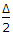# Civil Engineering - Surveying - Discussion

### Discussion :: Surveying - Section 8 (Q.No.2)

2.

If Δ is the angle of deflection of a simple curve of radius R, the length of its long chord, is

 [A]. R cos[B]. 2R cos[C]. R sin[D]. 2R sin.

Explanation:

No answer description available for this question.

 Sagar Naik said: (Apr 28, 2016) Why not option A?

 Abhishek Singh said: (Apr 2, 2017) It's option A.

 Abhishek said: (Apr 2, 2017) Please explain. Why not option A is correct?

 Rohit said: (Aug 31, 2017) Yes, Options D is right.

 Dhaval Vaghasiya said: (Jan 4, 2018) RcosΔ/2 = length between the midpoint of long chord and center of the curve. So, option D is right.

 Dave said: (Jun 4, 2018) Option D is correct. Considering the rigth angle on the center of the cord, then apply trigonometric functions.

 Jinish said: (Dec 21, 2019) Length of the chord - diameter multiply by the half degree of the sine. = D x sin angle/2 = 2R x sine θ/2